# 前端学数据结构与算法（十三）：01执行的艺术 - 回溯算法(上)

### 再次理解递归

#### 1480 - 一维数组的动态和 ↓

``````给你一个数组 nums , 请返回 nums 的动态和。

``````var runningSum = function (nums) {
for (let i = 0; i < nums.length - 1; i++) {
nums[i + 1] = nums[i + 1] + nums[i]
}
return nums
};

``````var runningSum = function (nums) {
const _helper = end => {
if (end === 0) { // 到数组头了，开始归
return nums
}
nums[end] = _helper(end - 1) + nums[end] // 它前面的元素加自身
return nums[end] // 返回计算后的结果
}
_helper(nums.length - 1) // 从最后一个数字开始
return nums
};

#### 22 - 括号生成 ↓

``````数字 n 代表生成括号的对数，请你设计一个函数，用于能够生成所有可能的并且有效的括号组合。

[
"((()))",
"(()())",
"(())()",
"()(())",
"()()()"
]

``````var generateParenthesis = function (n) {
const ret = [] // 存放有效的括号集合
const _helper = (left, right, parenthesi) => {
if (left === 0 && right === 0) { // 当左右括号的数量都用完时
ret.push(parenthesi) // 找到了一个有效的集合
}
if (left > 0) { // 放左括号的规则：当还有左括号时就可以进行放置
_helper(left - 1, right, parenthesi + '(') // 减少左括号的数量
}
if (right > left) { // 放右括号的规则：当右括号的剩余数量大于左括号时才能放置
_helper(left, right - 1, parenthesi + ')') // 减少右括号的数量
}
}
_helper(n, n, '') // 传入左括号和右括号的剩余数量
return ret
};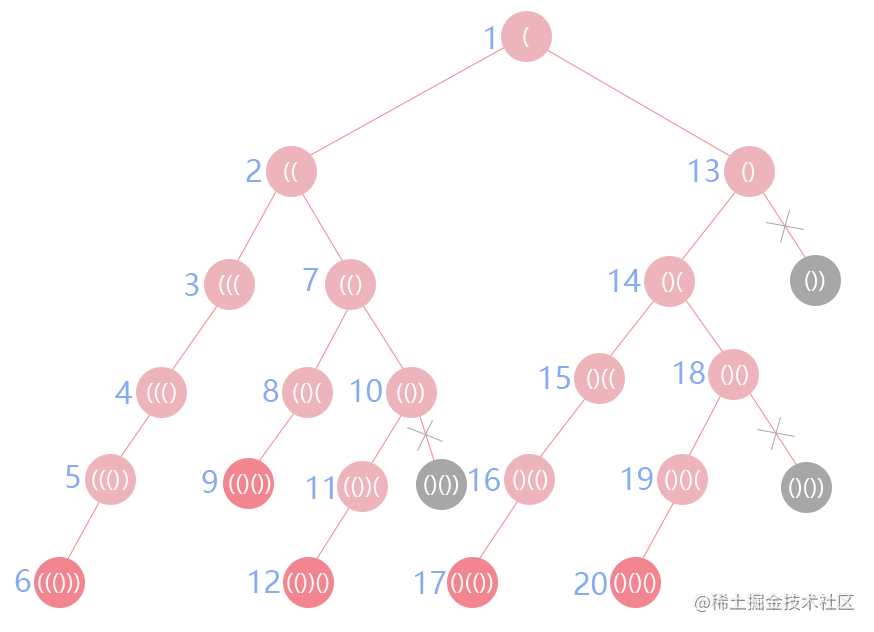### 组合问题

#### 17 - 电话号码的字母组合 ↓

``````给定一个仅包含数字 2-9 的字符串，返回所有它能表示的字母组合。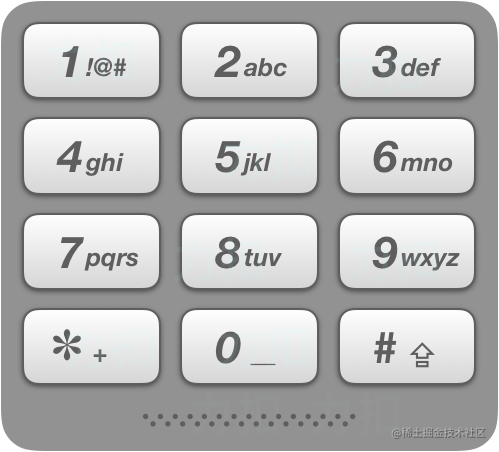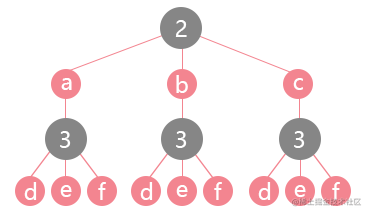``````const letterCombinations = digits => {
if (digits === '') { // 当输入空字符串时
return [] // 返回空集合
}
const ret = [] // 用于存放所有找到的组合
const letterArr = [' ', '', 'abc', 'def', 'ghi', 'jkl', 'mno', 'pqrs', 'tuv', 'wxyz']
// 存放数字对应的字母表

const _helper = (start, s) => { // 辅助函数
if (s.length === digits.length) { // 找到了一个组合
ret.push(s)
return // 返回上一层递归
}
const letters = letterArr[digits[start]] // 对应的就是abc, def
for (let i = 0; i < letters.length; i++) {
_helper(start + 1, s + letters[i]) // 执行多次递归
}
}

_helper(0, '')
return ret
};

#### 77 - 组合 ↓

``````给定两个整数 n 和 k，返回 1 ... n 中所有可能的 k 个数的组合。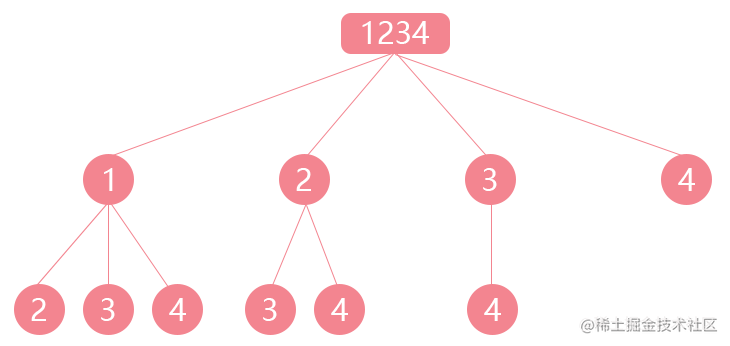``````var combine = function (n, k) {
if (n < 0 || k < 0 || k > n) { // n和k都得是符合条件的
return []
}
const ret = []
const _helper = (start, arr) => {
if (arr.length === k) {
ret.push(arr)
return
}
for (let i = start; i <= n; i++) { // i = start，忽略比start小的数进行组合
_helper(i + 1, arr.concat(i))
}
}
_helper(1, [])
return ret
};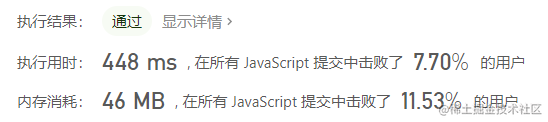``````_helper(i + 1, arr.concat(i))

``````var combine = function (n, k) {
if (n < 0 || k < 0 || k > n) {
return []
}
const ret = []
const _helper = (start, arr) => {
if (arr.length === k) {
ret.push([...arr]) // 需要进行拷贝
return
}
for (let i = start; i <= n; i++) {
arr.push(i)
_helper(i + 1, arr)
arr.pop()
}
}
_helper(1, [])
return ret
};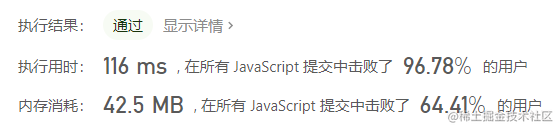#### 39 - 组合总和

``````给定一个无重复元素的数组 candidates 和一个目标数 target ，

candidates 中的数字可以无限制重复被选取。

所有数字（包括 target）都是正整数。
解集不能包含重复的组合。

``````var combinationSum = function (candidates, target) {
candidates.sort((a, b) => a - b)
const ret = []
const _helper = (start, sum, arr) => {
if (sum > target) { // 因为是排好序的，所以sum接下来只会更大
return
}
if (sum === target) { // 正好找到了
ret.push([...arr])
return
}
for (let i = start; i < candidates.length; i++) {
sum += candidates[i] // sum为计算结果
arr.push(candidates[i]) // arr存放暂存的集合
_helper(i, sum, arr) // 注意第一个参数没有+ 1，因为要无限使用
sum -= candidates[i]
arr.pop()
}
}
_helper(0, 0, [])
return ret
};